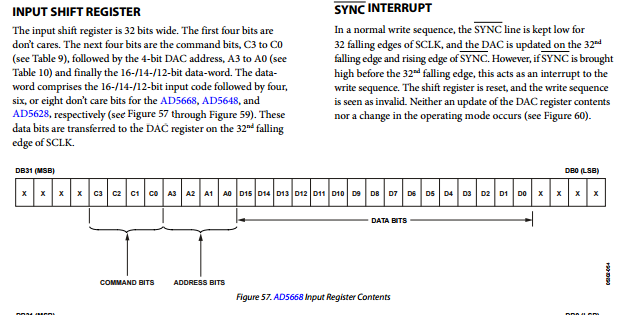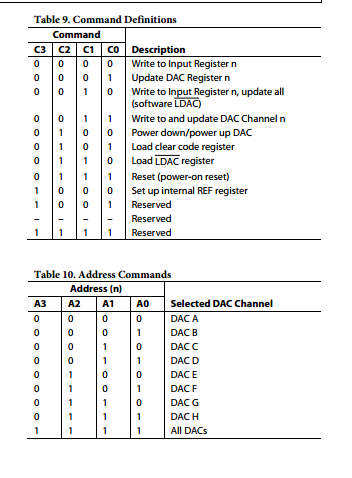## Trouble communication SPI

Hello, i want i send voltage to a component, which is regulated thanks to the AD5668. I wrote this code, but when i mesure the voltage, it kepps the same. Here is the code i wrote, maybe you could help me finding what's wrong :) Thanjks, Yann

```#include "mbed.h"
#include "limits.h"
Serial pc(USBTX, USBRX);
SPI spi(PA_7, PA_6, PA_5); // mosi, miso, sclk
DigitalOut cs(PA_4);

int main() {
int rep =0;
int bit32;
int ch;
int choix;

// Chip must be deselected

// Setup the spi for 8 bit data, high steady state clock,
// second edge capture, with a 1MHz clock rate
spi.format(16,1);
spi.frequency(1000000);

//-----------------------Conversion de l'entier en 16 bits----------------------
long int decimalNumber,remainder,quotient;
int i=1,j,temp;

pc.printf("Mettre a jour le registre ADC(2)\n\n");
pc.printf("Ecrire et mettre a jour le registre ADC(3)\n\n");
pc.printf("Mise sous tension ou hors tension(4)\n\n");
pc.printf("Reinitialiser la mise sous tension (5)\n\n");
scanf("%d",&ch);
switch(ch) {
case 01:
pc.printf("Quel pourcentage de voltage (0-65535) ?\n");
scanf("%ld",&decimalNumber);

quotient = decimalNumber;

while(quotient!=0){
temp = quotient % 16;

//To convert integer into character
if( temp < 10)
temp =temp + 48;
else
temp = temp + 55;

quotient = quotient / 16;
}

pc.printf("Equivalent hexadecimal value of decimal number %d: ",decimalNumber);
for(j = i -1 ;j> 0;j--)

//------------------------------------------------------------------------------
// Select the device by seting chip select low
cs = 0;
rep = spi.write(0x00FFFFF0);
wait(0.5);
cs=1;
pc.printf("\n rep: %d \n",rep);
break;
case 02:

cs=0;
rep = spi.write(0x01F00000);
wait(0.5);
cs=1;
pc.printf("\n rep2: %d \n",rep);
pc.printf("Modification effectuee \n");
break;

case 03:   pc.printf("Quel pourcentage de voltage (0-65535) ?\n");
scanf("%ld",&decimalNumber);

quotient = decimalNumber;

while(quotient!=0){
temp = quotient % 16;

//To convert integer into character
if( temp < 10)
temp =temp + 48;
else
temp = temp + 55;

quotient = quotient / 16;
}

pc.printf("Equivalent hexadecimal value of decimal number %d: ",decimalNumber);
for(j = i -1 ;j> 0;j--)

// Select the device by seting chip select low
cs = 0;
spi.write(0x0);
rep = spi.write(0x02FFFFF0);
wait(0.5);
cs=1;
pc.printf("\n rep: %d \n",rep);
break;
case 04:  pc.printf("Souhaitez vous une mise en tension(1) ou hors tension (2) ?\n");
scanf("%d",&choix);
if (choix == 1) {
pc.printf("bien recu m\n");
}
else if (choix == 2) {
pc.printf("bien recu h\n");
} else {
pc.printf("Commande non reconnue\n");
}
break;
default: break;
}

}
```Here is the method to write to the AD. Do we agree that 0x02FFFFF0 should set the maximum voltage value et apply it directly ?

I just tried to mesure the voltage again and by changing the lines, i succeeded in getting a 7.12V voltage (started at 1). But i sent the maximum 16 bit value (FFFF) and it doesn't get me the maximum voltage, why ?

``` // Select the device by seting chip select low
cs = 0;
spi.write(0x02);
spi.write(0xFF);
spi.write(0xA5);
spi.write(0xF0);

//wait(0.5);
cs=1;
pc.printf("\n rep: %d \n",rep)
```
posted by Yann De Baudus 09 Mar 2017

##### 4 years, 4 months ago.

You need to break your write up into two 16-bit transactions, or four 8-bit transactions in order to shift all 32 bits required. Make sure you shift the most significant byte or word first. (EDIT: I see you are trying something like this. Make sure you set the format back to 8 bits though if you are doing 4 transactions)

Thank you for your answer as you said, i tried it and it seems to work, but nos as correctly as it should ! How do you make sure you shift the most significant byte or word first ? And do i have to set the format at 16 or 32 ?

posted by Yann De Baudus 09 Mar 2017

I would keep the format as 8 bit

```spi.format(8,1);
```

and then shift the value out byte by byte such as

```....
unsigned int val = 0xAABBCCDD; //whatever your 32-bit value to be written is
....
spi.write((uint8_t)val>>24);
spi.write((uint8_t)val>>16)
spi.write((uint8_t)val>>8);
spi.write((uint8_t)val);
...
```

I have not looked at your algorithm in detail but make sure you follow all of the required initialization in the datasheet.

Bill

posted by Bill Bellis 10 Mar 2017

So i just tried your method, and it doesn't seem to work, the voltage is still the same ? Is it possible to do it like below ?

```spi.write((uint16_t)val>>16);

spi.write((uint16_t)val);
```

So i choose format (16,1) Plus, do i have to send bits in order to make the AD understand i want to "talk" to him before sending bit stream ? I am a bit confused

posted by Yann De Baudus 10 Mar 2017
##### 4 years, 4 months ago.

The code above is very hard to read and understand. Please use the `<<code>> and <</code>>` tags to post your code.

Looks like you are setting up SPI in 16 bit mode

```spi.format(16,1);
```

However, you send values as 32 bit. The SPI write will truncate that to the least significant 16 bits.

```//Select the device by seting chip select low
cs = 0;
rep = spi.write(0x00FFFFF0);
wait(0.5);
cs=1;
```

Thank you for your answer, i corrected it, but the datasheet says it is a 16 bits SPI voltage output, BUT they ask me 32 bits to communicate with the AD5668 (4 useless, 4 command, 4 adress, 16 instruction, 4 useless again) :/

posted by Yann De Baudus 09 Mar 2017